Sine -Gordon Solitons and Soliton Collisions:
Elastic Ribbon Model

by

Andrey E. Miroshnichenko,  andrey.miroshnichenko@anu.edu.au,

Aleksey A. Vasiliev,  aleksey.vasiliev@tversu.ru,

Sergey V. Dmitriev, sergey@iis.u-tokyo.ac.jp

NOTE: We use Maple for derivation of the one-, two- and three-soliton solutions to the sine-Gordon equation. With the use of Maple animation tools the solutions are visualized in frame of the elastic ribbon model.

Introduction

The basic properties of solitons, like propagation and interaction without change in their velocity and shape, make it possible to treat them as the robust localized objects. Solitons show their duality, having both the properties of particles and waves. A soliton has the wave nature and finite width but it behaves itself as a particle when interacting with other solitons. That is why the solitons are often spoken of as quasiparticles. It is known that for solitons in a completely integrable system there exist an infinitely large number of conservation laws. For example, not only the energy and momentum but also a characteristic, which can be called "charge", is conserved in the system. There exist several physical interpretations of the sine-Gordon equation, therefore this charge will be called here the topological charge, without specification for a particular model.

Elastic Ribbon Model

Let us twist one end of the elastic ribbon with respect to another through angle 2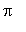. As a result of this operation a twist, which can propagate along the ribbon, will be formed Ref. . The ribbon can be twisted either clockwise or counterclockwise. Therefore, the twist can be regarded as a charged particle and the sign of charge is defined by the direction of twisting.

 > restart:

 > with(plots):

 > with(plottools):

```Warning, the name changecoords has been redefined

```

```Warning, the assigned name arrow now has a global binding

```

Procedure "ribbon" visualizes the solutions to the sine-Gordon equation for the elastic ribbon model.

Input parameters:
N_Sol - N-soliton sine-Gordon solution,
name - name of the solution,
L0, L1 - space domain for visualization,
T0, T1 - time domain,
orient - point of view on the system.

 > ribbon:=proc(N_Sol,name,L0,L1,T0,T1,orient)

 >

 > assume(nt,integer);assume(ndx,integer);

 > assume(nx,integer);assume(nxx,integer);

 > nt:=40: ndx:=10:

 > nx:=10: nxx:=nx*ndx:

 > L:=L1-L0: T:=T1-T0:

 > dx:=L/nxx: dt:=T/nt:

 >

 > bases_line:=line([0,0,0],[L,0,0],color=black );

 > for j from 0 to nxx+1 do

 > dd[j]:=dx*j;

 > od:

 > for i from 0 to nt do

 > for j from 1 to nxx do

 > theta:=N_Sol(L0+dd[j],T0+i*dt)+Pi/2;

 > od:

 > for j from 1 to nx do

 > j1:=j*ndx;

 > arrows[j]:=arrow([dd[j1],0,0],[dd[j1],cos_u[j1],sin_u[j1]],tar,3*tar, 0.2,color=blue);

 > od;

 > seq_arrows:=seq(arrows[j],j=1..nx):

 > for j from 1 to nxx-1 do

 > lines[j]:=line([dd[j],cos_u[j],sin_u[j]],[dd[j+1],cos_u[j+1],sin_u[j+1]],color=red,thickness=2);

 > od;

 > seq_lines:=seq(lines[j],j=1..nxx-1):

 > for j from 1 to nxx+1 do

 > theta_r:=N_Sol(L0+dd[j],T0+i*dt);

 > od;

 > for j from 2 to nxx+1 do

 > polygons[j]:=polygon([[dd[j-1],-cos_u_r[j-1],-sin_u_r[j-1]],[dd[j],-cos_u_r[j],-sin_u_r[j]],[dd[j-1],cos_u_r[j-1],sin_u_r[j-1]],[dd[j],cos_u_r[j],sin_u_r[j]]], color=green); od:

 > seq_polygons:=seq(polygons[j],j=2..nxx+1);

 > b[i]:=display([seq_arrows,seq_lines,bases_line,seq_polygons], color=red):

 > od:

 > display(seq(b[i],i=0..nt), insequence=true,axes=framed,title=name,scaling=unconstrained,orientation=orient);

 > end:

Soliton Solutions

Hierarchy of sine-Gordon multi-soliton solutions can be obtained by means of Backlund transformations Ref. . Procedure "Backlund" realizes the algorithm of Backlund transformation in Maple V.
Input parameters:
a1, a2 - parameters of transformation,
phi0 - (N-2)-soliton solution,
phi1, phi2 - two (N-1)-soliton solutions.
The outcome of this procedure is the N-soliton solution.

 > Backlund := proc (a1,a2,phi0,phi1,phi2) RETURN(phi0+4*arctan(((a1+a2)*tan((phi1-phi2)/4))/(a1-a2))) end;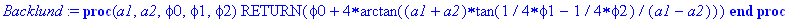Procedure "Soliton" builds a single-soliton solution.
Input parameters:
x, t - space and time coordinates,
a - parameter of transformation,
x_0 - initial position.

 > Soliton := proc (x,t,a,x_0) local xi,tau: xi:=(x-x_0+t)/2; tau:=(x-x_0-t)/2; RETURN(4*arctan(exp(a*xi+tau/a))) end;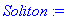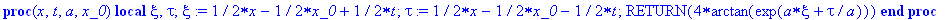Procedure "TwoSoliton" builds the two-soliton solutions.
Input parameters:
x, t - space and time coordinates,
a1, a2 - parameters of transformation,
x_1, x_2 - initial positions.

 > TwoSoliton := proc (x,t,a1,x_1,a2,x_2) local phi0,phi1,phi2: phi0:=(x,t)->0; phi1:=(x,t)->Soliton(x,t,a1,x_1); phi2:=(x,t)->Soliton(x,t,a2,x_2); phi1:=(x,t)->Backlund(a1,a2,phi0(x,t),phi1(x,t),phi2(x,t)); RETURN(phi1(x,t))  end;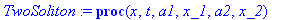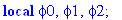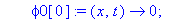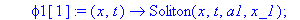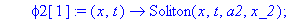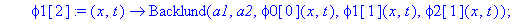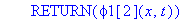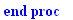Procedure "ThreeSoliton" builds the three-soliton solutions.
Input parameters:
x, t - space and time coordinates,
a1, a2, a3 - parameters of transformation,
x_1, x_2, x_3 - initial positions.

 > ThreeSoliton := proc (x,t,a1,x_1,a2,x_2,a3,x_3) local  phi,phi1,phi2,phi3 : phi2:=(x,t)->Soliton(x,t,a2,x_2); phi1:=(x,t)->TwoSoliton(x,t,a1,x_1,a2,x_2); phi2:=(x,t)->TwoSoliton(x,t,a2,x_2,a3,x_3); phi1:=(x,t)->Backlund(a3,a1,phi2(x,t),phi2(x,t),phi1(x,t)); RETURN(Re(phi1(x,t)))  end;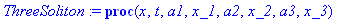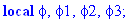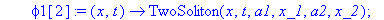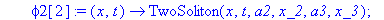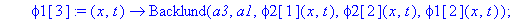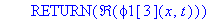One-Soliton Solutions

Kink

 > v[K]:=0.1:                   # velocity of the Kink

 > x_K:=-4*sqrt(1-v[K]^2):      # initial state

 > a:=sqrt((1-v[K])/(1+v[K])):  # parameter of transformation

 > L:=40*sqrt(1-v[K]^2): T:=(L-2*x_K)/v[K]:

 > K:=(x,t)->Soliton(x,t,a,x_K):

 > orient:=[115,65]:

 > ribbon(K,"Kink",0,L,0,T,orient);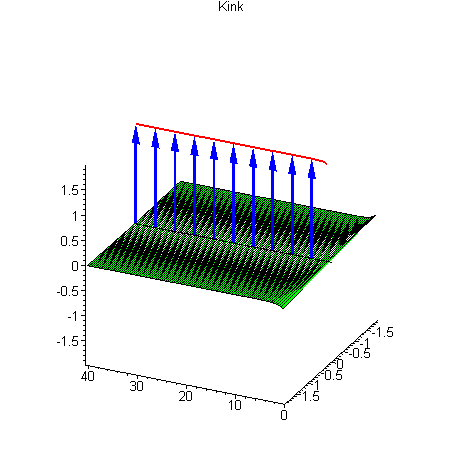It is seen from the figure that the kink solution describes the twist obtained by the counterclockwise twisting of the ribbon. Hence, the kink has the charge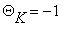.

AntiKink

 > v[AK]:=0.1:                    # velocity of the AntiKink

 > x_AK:=-4*sqrt(1-v[AK]^2):      # initial state

 > a:=-sqrt((1-v[AK])/(1+v[AK])): # parameter of transformation

 > L:=40*sqrt(1-v[AK]^2): T:=(L-2*x_AK)/v[AK]:

 > AK:=(x,t)->Soliton(x,t,a,x_AK):

 > orient:=[115,65]:

 > ribbon(AK,"AntiKink",0,L,0,T,orient);

 >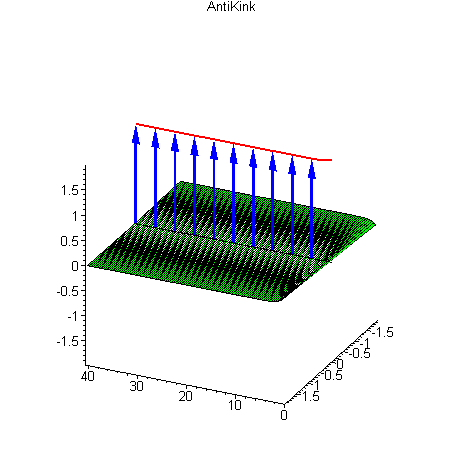The antikink solution describes the twist obtained by the clockwise twisting of the ribbon. Antikink has the charge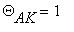.

Two-Soliton Solutions

Kink-Kink Collision

 > v[K_K]:=0.2:    # velocity of the Kink-Kink

 > x_K_K:=0:       # initial state

 > a:=sqrt((1-v[K_K])/(1+v[K_K])):  # parameters of

 > a:=-sqrt((1+v[K_K])/(1-v[K_K])): # transformation

 > L:=10/sqrt(1-v[K_K]^2): T:=L/v[K_K]:

 > K_K:=(x,t)->TwoSoliton(x,t,a,x_K_K,a,x_K_K):

 > orient:=[115,65]:

 > ribbon(K_K,"Kink-Kink Collison",-3*L,3*L,-4*T,4*T,orient);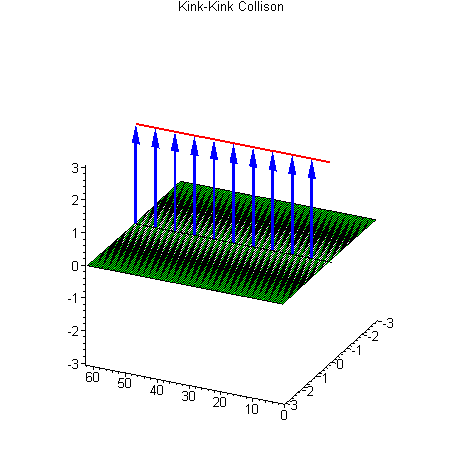At the initial time the two kinks are widely spaced and move toward each other. It can be seen that kinks pass through each other without any changes. In this example the two twists propagate in the ribbon and the total charge is conserved. The total charge of the kink-kink pair is equal to the sum of charges,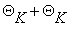, so that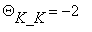.

Kink-AntiKink Collision

 > v[K_AK]:=0.2:   # velocity of the Kink-AntiKink

 > x_K_AK:=0:      # initial state

 > a:=sqrt((1-v[K_AK])/(1+v[K_AK])): # parameters of

 > a:=sqrt((1+v[K_AK])/(1-v[K_AK])): # transformation

 > L:=10/sqrt(1-v[K_AK]^2): T:=L/v[K_AK]:

 > K_AK:=(x,t)->TwoSoliton(x,t,a,x_K_AK,a,x_K_AK):

 > orient:=[115,65]:

 > ribbon(K_AK,"Kink-AntiKink Collision",-3*L,3*L,-4*T,4*T,orient);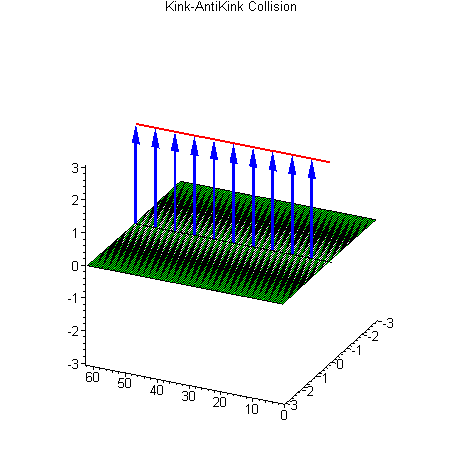Colliding kink and antikink also do not suffer any changes. The total charge of the kink-antikink pair is equal to the sum of charges,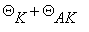, so that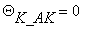. During the kink-antikink collision, at a certain moment, the two twists disappear.

Breather Solutions

Standing Breather

 > v[B]:=0:       # velocity of the Breather

 > omega:=0.154:  # frequency of the Breather

 > x_B:=0:        # initial state

 > R:=sqrt((1-v[B])/(1+v[B])):

 > eta:=sqrt(1-omega^2):

 > a:=R*(eta+I*omega):  # parameters of

 > a:=R*(eta-I*omega):  # transformation

 > T:=evalf(2*Pi/omega):

 > L:=evalf(4*arctan(eta/omega)):

 > B:=(x,t)->TwoSoliton(x,t,a,x_B,a,x_B):

 > orient:=[115,65]:

 > ribbon(B,"Standing Breather",-2*L,2*L,-T,T,orient);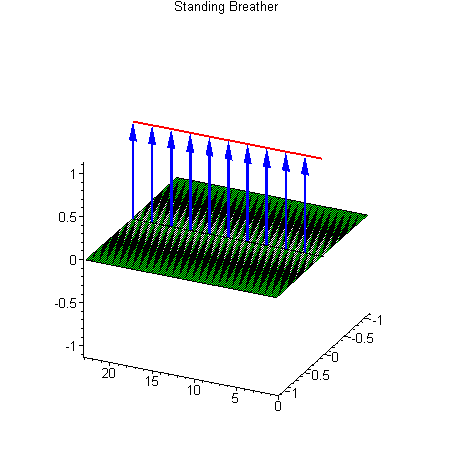In this figure the so-called breather solution, which is one of the most beautiful sine-Gordon solutions, is presented. Note that the ends of the ribbon are not twisted one with respect to another. Consequently, the charge of the breather is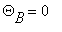which coincides with the charge of the kink-antikink pair. This coincidence is not accidental, because the breather actually is the kink and antikink bound together in an oscillatory system.

Large amplitude Breather

 > v[B]:=0.2:      # velocity of the Breather

 > omega:=0.154:   # frequency of the Breather

 > x_B:=0:         # initial state

 > R:=sqrt((1-v[B])/(1+v[B])):

 > eta:=sqrt(1-omega^2):

 > a:=R*(eta+I*omega):  # parameters of

 > a:=R*(eta-I*omega):  # transformation

 > T:=evalf(8*Pi/omega):

 > L:=evalf(T*abs(v[B])/(sqrt(1-v[B]^2))):

 > B:=(x,t)->Re(TwoSoliton(x,t,a,x_B,a,x_B)):

 > orient:=[115,65]:

 > ribbon(B,"Large amplitude Breather",0,L,0,T,orient);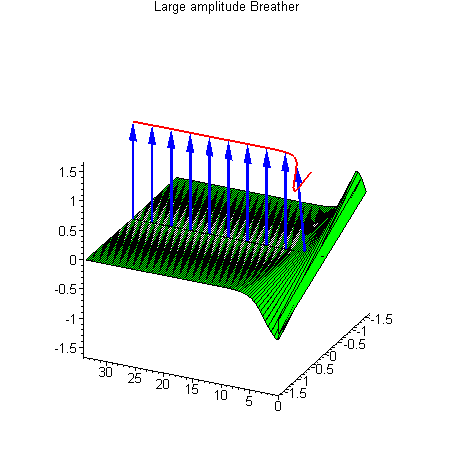The moving breather looks attractive as well.

Small amplitude Breather

 > v[B]:=0.85:    # velocity of the Breather

 > omega:=0.99:   # frequency of the Breather

 > x_B:=0:        # initial state

 > R:=sqrt((1-v[B])/(1+v[B])):

 > eta:=sqrt(1-omega^2):

 > a:=R*(eta+I*omega):  # parameters of

 > a:=R*(eta-I*omega):  # transformation

 > T:=evalf(2*2*Pi/omega):

 > L:=evalf(T*abs(v[B])/(sqrt(1-v[B]^2))):

 > B:=(x,t)->Re(TwoSoliton(x,t,a,x_B,a,x_B)):

 > orient:=[111,42]:

 > ribbon(B,"Small amplitude Breather",-2*L,2*L,-4*T,4*T,orient);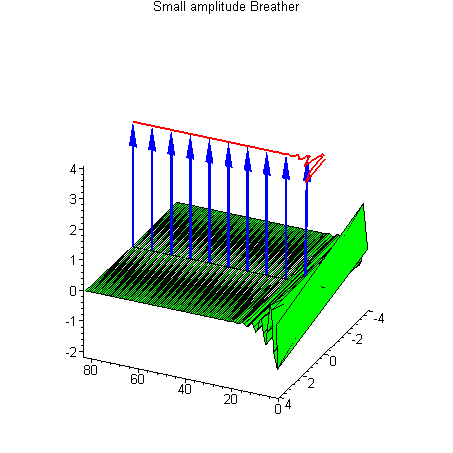This is also the breather. It resembles the ripple of a water surface.

Three-Soliton Solutions

Standing Kink and Moving Breather collision

 > v[K]:=0:       # velocity of the Kink

 > v[B]:=0.5:     # velocity of the Breather

 > omega:=0.154:  # frequency of the Breather

 > R:=sqrt((1-v[B])/(1+v[B])):

 > eta:=sqrt(1-omega^2):

 > a:=R*(eta+I*omega):           # parameters of

 > a:=sqrt((1-v[K])/(1+v[K])):   # transformation

 > a:=R*(eta-I*omega):

 > T:=evalf(2*Pi/omega):

 > L:=evalf(T*abs(v[B])/(sqrt(1-v[B]^2))):

 > x_K:=L/2:      # initial state of the Kink

 > x_B:=0:        # initial state of the Breather

 > K_B:=(x,t)->ThreeSoliton(x,t,a,x_B,a,x_K,a,x_B):

 > orient:=[105,65]:

 > ribbon(K_B,"Standing Kink and Moving Breather collision",-0.5*L,1.5*L,-T,2*T,orient);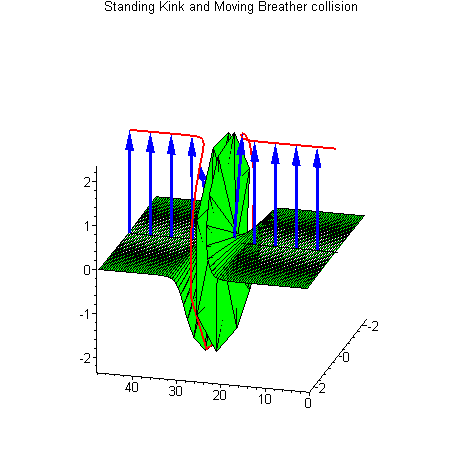Standing Breather and Moving Kink collision

 > v[K]:=0.5:     # velocity of the Kink

 > v[B]:=0:       # velocity of the Breather

 > omega:=0.154:  # frequency of the Breather

 > R:=sqrt((1-v[B])/(1+v[B])):

 > eta:=sqrt(1-omega^2):

 > a:=R*(eta+I*omega):         # parameters of

 > a:=sqrt((1-v[K])/(1+v[K])): # transformation

 > a:=R*(eta-I*omega):

 > L:=evalf(10/sqrt(1-v[K]^2)):

 > x_K:=-4*sqrt(1-v[K]^2):  # initial state of the Kink

 > x_B:=L/2:                # initial state of the Breather

 > T:=evalf((L-2*x_K)/v[K]):

 > K_B:=(x,t)->ThreeSoliton(x,t,a,x_B,a,x_K,a,x_B):

 > orient:=[-80,77]:

 > ribbon(K_B,"Standing Breather Moving Kink collision",-L,3*L,-T,2*T,orient);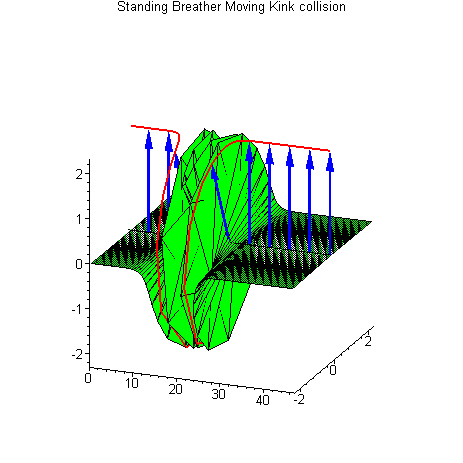In the two last figures, the kink-breather collisions are presented for the cases when one of the quasiparticles moves and another one is at rest. Of course, for these collisions the total charge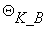=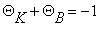, is also conserved. These two solutions, describing kink-breather collisions, are actually identical in the sense that one of them can be transformed to another by means of the Lorentz transformation for an inertial system. All the collisions described above are the elastic collisions or, in other words, there is no energy and momentum exchange between colliding quasiparticles. However, for the discrete sine-Gordon system the collision of more than two solitons can be strongly inelastic even at a very small degree of discreteness. For example, in Ref. , the domain of parameters has been given where the transformation of kink-breather solution to the kink-antikink-kink solution becomes possible. This transformation is not forbidden by the charge conservation law because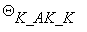=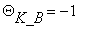.

Conclusion

In the present worksheet, with the use of MAPLE, the multi-soliton solutions to the sine-Gordon equation were discussed in the frame of the elastic ribbon model, which allows the consideration of solitons as the charged particles. For the presented animations we tried to choose the parameters in a way to demonstrate, in the clearest form, the charge conservation law.

Disclaimer

While every effort has been made to validate the solutions in this worksheet, Waterloo Maple Inc. and the contributors are not responsible for any errors contained and are not liable for any damages resulting from the use of this material

References

 R.K.Dodd, J.C.Eilbeck, J.D.Gibbon, H.C.Morries, Solitons and Nonlinear Wave Equations (Academic Press, London, 1982).

 S.V.Dmitriev, T.Shigenari, A.A.Vasiliev, A.E.Miroshnichenko, Effect of  discreteness on a sine-Gordon three-soliton solution // Phys.Lett. A   246 (1998) 129-134.